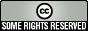# News You Can Bruise for 2007January 18

: This is even cooler than the Mandelbrot generator. Via clickolinko I found this excellent function which you shouldn't click just yet because I've got a more entertaining presentation of it. You're supposed to graph the function

1/2 < |_mod(|_y/17_| 2-17|_x_|-mod(|_y_|,17),2)_|
over a certain range. Here's a Python program that does it:
```#!/usr/bin/python
from dmath import getcontext, Decimal, floor
getcontext().prec = 550

# Tweak these to get a larger or smaller-scale graph.
X_STEP = Decimal("1")
Y_STEP = X_STEP

# Don't mess with these.
X_MIN = Decimal(0)
X_MAX = X_MIN + 105
Y_MIN = Decimal("96093937991895888497167296212785275471500433" +
"96601293066515055192717028023952664246896428" +
"42174350718121267153782770623355993237280874" +
"14430789132596394133772348785773574982392662" +
"97155171737169951652328905382216124032388558" +
"66184013235585136048828693337902491454229288" +
"66708109618449609170518345406782773155170540" +
"53816273809676025656250169814820834187831638" +
"49115590225610003652351370343874461848378737" +
"23819822484986346503315941005497470059313833" +
"92264972494617515457283667023697454610146559" +
"97933798537483143786841806593422227898388722" +
"980000748404719")
Y_MAX = Y_MIN + 16

fl = floor
# Performance speedup
FLOORS_DIV_17 = {}
def fl17(n):
"A memoized floor(n/17) function."
f = FLOORS_DIV_17.get(n)
if not f:
f = fl(n/17)
FLOORS_DIV_17[n] = f
return f

half = Decimal(".5")
def f(x,y):
return half < fl((((fl17(y))*(2**((-17*fl(x)) - (fl(y) % 17)))) % 2))

y = Y_MIN
while y <= Y_MAX:
x = X_MAX
while x >= X_MIN:
if f(x,y):
print "*",
else:
print " ",
x = x - X_STEP
print
y = y + Y_STEP
```

It uses the dmath library so I can call the `floor` function on an arbitrary-precision decimal. Anyway, you can run that program yourself or you can see my premade ASCII graph of the function If you graph the function over other ranges you can see other patterns, but none so striking.

Lots more interesting empirical math in this paper.

[Main]Unless otherwise noted, all content licensed by Leonard Richardsonunder a Creative Commons License.